# Conley index

(diff) ← Older revision | Latest revision (diff) | Newer revision → (diff)

A tool to analyze the dynamics of continuous or discrete dynamical systems. It can be used, for instance, to find special orbits like stationary, periodic or heteroclinic orbits, or to prove chaotic behaviour of the system. It has been applied to a wide range of problems, e.g. to find travelling-wave solutions of partial differential equations; to investigate the structure of global attractors of reaction-diffusion equations or delay equations; to find periodic solutions of Hamiltonian systems; to give a rigorous computer-assisted proof of chaos in Lorenz equations; to prove bifurcation and to analyze the set of bifurcating solutions in various settings. The original work of C. Conley and his school took place in the 1970s and early 1980s. Standard references for this work are [a3], [a12] and [a4]. A recent overview on the Conley index and its applications is [a8].

In order to describe the basic version of the Conley index, consider a flowon a locally compact metric space(cf. also Flow (continuous-time dynamical system)). A compact subsetis called isolated invariant if there exists a compact neighbourhoodofinsuch thatis the invariant part of: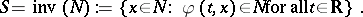In that caseis said to be an isolating neighbourhood of. The Conley index associates to an isolated invariant setthe homotopy type of a pointed topological space in the following way. An index pair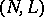for an isolated invariant setconsists of compact subsets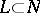ofsuch that:

i)is an isolating neighbourhood of;

ii)is positively invariant in: Given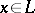and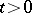with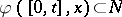, then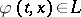;

iii)is an exit set for: Given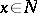andwith, there exists a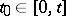with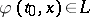. Given an isolated invariant set, it can be proved that index pairs exist. Moreover, ifand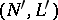are two index pairs for, then the quotient spaces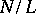and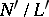are homotopy equivalent with base pointsand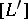fixed (cf. also Homotopy). The Conley index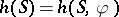ofis by definition the homotopy type of the pointed space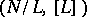, whereis an index pair for.

As an example, consider the flow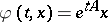on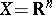, where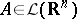has no eigenvalues on the imaginary axis; e.g.with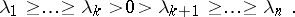The origin is a hyperbolic stationary point of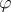andis an isolated invariant set. Any compact neighbourhood ofis an isolating neighbourhood of. Suppose that the generalized eigenspace ofcorresponding to the eigenvalues with positive real part is spanned by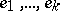, and the complementary generalized eigenspace is spanned by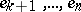. Then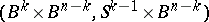is an index pair for; hereis the unit ball in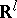with boundary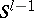. Sinceis contractible (cf. also Contractible space), the Conley index ofis equal to the homotopy type of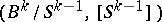, which is the same as the homotopy type of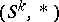.

In this example one recovers the Morse index of the hyperbolic fixed point. Therefore the Conley index can be interpreted as a generalized Morse index. In applications one usually first has a setwhich is an isolating neighbourhood of some a priori unknown isolated invariant set. Then one tries to compute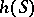or to obtain some information, like its homology groups. For this computation the invariance of the Conley index under certain deformations of the flow, the continuation invariance, is very useful — in analogy to the homotopy invariance of the Brouwer degree. Finally one can use the knowledge aboutin order to investigate the invariant setitself. Whereas one can immediately deduce thatis not empty ifis not trivial, additional information on the flow insideis needed in order to obtain more detailed results about, for example thatcontains a periodic orbit.

The original version of the Conley index has been refined and extended in several directions. For an equivariant version together with a product structure on the cohomology level, see [a6]. The Conley index and this additional structure played an important role in Floer's work on the Arnol'd conjecture and in the development of Floer homology. In [a11] and [a2] the Conley index has been generalized to semi-flows on metric spaces which need not be locally compact. This has been applied to parabolic differential equations and delay differential equations. Discrete dynamical systems are being considered in [a10] and [a9], multi-valued discrete dynamical systems in [a7]. The multi-valued version is the basis for rigorous numerical computations of the Conley index for concrete dynamical systems, since it allows one to incorporate interval arithmetic. Parametrized versions of the Conley index have been defined in [a1] and [a5]; an abstract categorical approach is given in [a13].

How to Cite This Entry:
Conley index. Encyclopedia of Mathematics. URL: http://encyclopediaofmath.org/index.php?title=Conley_index&oldid=14232
This article was adapted from an original article by Thomas Bartsch (originator), which appeared in Encyclopedia of Mathematics - ISBN 1402006098. See original article